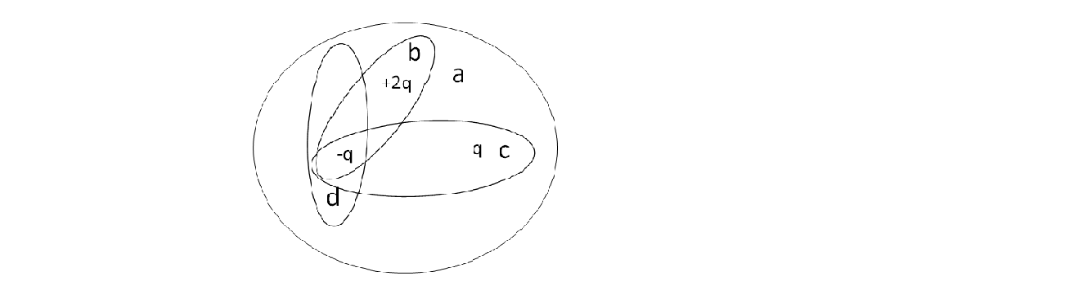# Problem: The figure shows four Gaussian surfaces surrounding a distribution of charges. Which Gaussian surfaces have no electric flux through them? (Check all that apply).(a) d(b) a(c) b(d) c

80% (90 ratings)
###### Problem Details

The figure shows four Gaussian surfaces surrounding a distribution of charges. Which Gaussian surfaces have no electric flux through them? (Check all that apply).

(a) d

(b) a

(c) b

(d) cFrequently Asked Questions

What scientific concept do you need to know in order to solve this problem?

Our tutors have indicated that to solve this problem you will need to apply the Gauss' Law concept. You can view video lessons to learn Gauss' Law. Or if you need more Gauss' Law practice, you can also practice Gauss' Law practice problems.

How long does this problem take to solve?

Our expert Physics tutor, Juan took 1 minute and 25 seconds to solve this problem. You can follow their steps in the video explanation above.

What professor is this problem relevant for?

Based on our data, we think this problem is relevant for Professor Efthimiou's class at UCF.